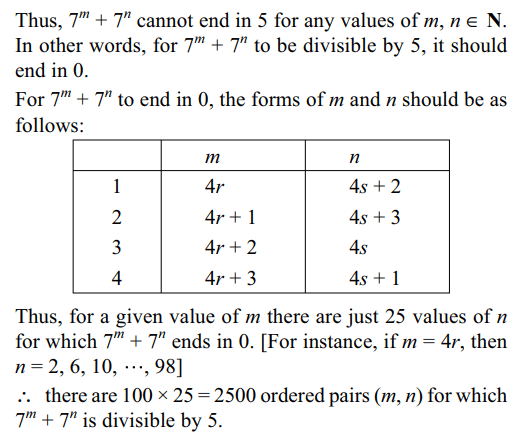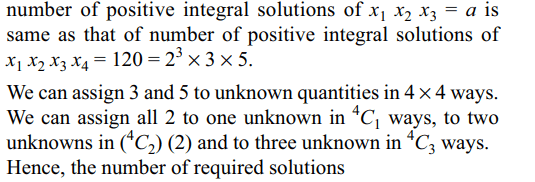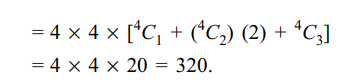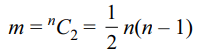## Permutations and Combinations Questions and Answers Part-5

1. Sum of all three digit numbers (no digit being zero) having the property that all digits are perfect squares, is
a) 3108
b) 6216
c) 13986
d) 310

Explanation: The non-zero perfect square digits are 1, 4 and 9.
1 can occur at units place in 3 × 3 = 9 ways.
Sum due to 1 at unit’s place is 1 × 9. Similarly, sum due to 1 at ten’s place is 1 × 10 × 9 and sum due to 1 at hundred’s place is 1 × 100 × 9. We can deal with the digits 4 and 9 in a similar way.
Thus, sum of the desired number is
(1 + 4 + 9) (1 + 10 + 100) (9) = 13986

2. The number of ordered pairs $\left(m,n\right),m,n \epsilon \left\{1,2,....,100\right\}$      such that $7^{m}+7^{n}$   is divisible by 5 is
a) 1250
b) 2000
c) 2500
d) 5000

Explanation: Note that 7r (r ∈ N) ends in 7, 9, 3 or 1 (corresponding to r = 1, 2, 3 and 4 respectively).3. The greatest value of $^{2n}C_{r}\left(0\leq r\leq2n\right)$     is
a) $^{2n}C_{n-1}$
b) $^{2n}C_{n+1}$
c) $^{2n}C_{n}$
d) $^{2n}C_{n-2}$

Explanation:4. Let $a=\sum_{i< j}\left(\frac{1}{^{n}C_{i}}+\frac{1}{^{n}C_{j}}\right)$     and $b=\sum_{i< j}\left(\frac{i}{^{n}C_{i}}+\frac{j}{^{n}C_{j}}\right)$     , then
a) $b=\left(n-1\right)a$
b) $b=\left(n+1\right)a$
c) $b=\frac{n}{2}a$
d) $b=n-a$

Explanation:5. Let S = {1, 2, 3, .... , n}. If X denote the set of all subsets of S containing exactly two elements, then the value of $\sum_{A \epsilon X}$ (min A) is
a) $^{n+1}C_{3}$
b) $^{n}C_{3}$
c) $^{n}C_{2}$
d) $^{n+1}C_{4}$

Explanation: There are exactly (n – 1) subsets of S containing two elements having 1 as least element; exactly (n – 2) subsets of S having 2 as least element and so on. Thus,6. Let 'a' be a factor of 120, then the number of positive integral solutions of $x_{1}x_{2}x_{3}=a$   is
a) 160
b) 320
c) 480
d) 960

Explanation: Let x4 be such that x4 = 120/a. Then, the7. The value of $n\epsilon N$   for which $^{m}C_{2}=210$     where $m=^{n}C_{2}$    is
a) 4
b) 5
c) 6
d) 7

Explanation:8. A committee consisting of at least three members is to be formed from a group of 6 boys and 6 girls such that it always has a boy and a girl. The number of ways to form such committee is:
a) $2^{12}-2^{7}-13$
b) $2^{11}-2^{6}-13$
c) $2^{12}-2^{7}-35$
d) $2^{11}-2^{7}-35$

Explanation:9. The total number of ways in which 5 balls of different colours can be distributed among 3 persons so that each person gets at least one ball is
a) 75
b) 150
c) 210
d) 243